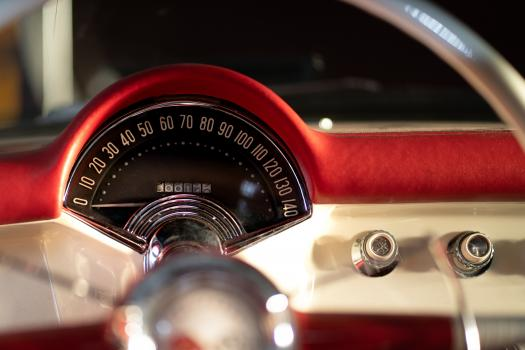# Instantaneous Rate Of Change

5 Questions | Total Attempts: 614SettingsCreate your own QuizCan you interpret and/or estimate instantaneous rate of change in multiple representations?

• 1.
The graph shows y = f(x) in purple. Which line has a slope equal to the rate expression shown?
• A.

Line a

• B.

Line b

• C.

Line c

• D.

Line d

• 2.
Estimate the instantaneous rate using the graph of y = f(x).
• A.

0.02

• B.

–0.02

• C.

7.25

• D.

–7.25

• 3.
Acceleration is the rate of change of velocity. If the velocity of a car driving on a straight road is given by v(t), which of the following expressions represent the car's instantaneous expression at t = 5 seconds? (Check all that apply.)
• A.

Expression 1:

• B.

Expression 2:

• C.

Expression 3:

• D.

Expression 4:

• E.

Expression 5:

• F.

Expression 6:

• 4.
Estimate the instantaneous rate of change of H(x) = sin(ln x2) at x = –3
• A.

0.1

• B.

0.4

• C.

0.8

• D.

3.5

• E.

Undefined

• 5.
Which statement(s) correctly contrast average rate of change and instantaneous rate of change?
• A.

Average rate of change requires specifying two points on a graph while instantaneous rate of change requires only one.

• B.

Average rate of change can be interpreted as a slope while instantaneous rate of change can not.

• C.

Average rate of change can be for any function while instantaneous rate of change is only for nonlinear functions.

• D.

Average rate of change can be calculated as a difference quotient while instantaneous rate of change is a limit of a difference quotient.

Related TopicsBack to top
×

Wait!
Here's an interesting quiz for you.